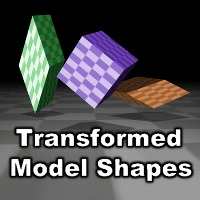# Transformed Model Shapes

#### Greenblizzard

You must be logged in to obtain assets

### Description

Add pretransformed and coloured basic shapes to d3d models!

Description: A set of scripts to add transformed basic shapes to d3d models. The scripts mimic the built-in d3d_model_block/ellipsoid/floor/cone() etc. functions, but unlike these, are affected by the 3D transformation. This allows a single d3d model to consist of several shapes with different static transformations.

The scripts also take two optional arguments to make the shapes have their own colour and alpha values, giving room for even more variation.

(!)While the built-in shape functions add single-line objects to the model data, which merely describe the shape, the shapes added by these scripts are triangle list primitives, so expect a bigger file size if you save the model with d3d_model_save().

Scripts:

• tr3d_init()
• tr3d_matrix_update()
• tr3d_transform_point()
• tr3d_transform_normal()
• tr3d_model_floor()
• tr3d_model_wall()
• tr3d_model_block()
• tr3d_model_cylinder()
• tr3d_model_cone()
• tr3d_model_ellipsoid()

Example code:

``````tr3d_init():
model = d3d_model_create();
d3d_transform_set_rotation_x(45);
tr3d_model_cone(model,-5,-5,-5,5,5,5,1,1,true,24); // Rotated cone
tr3d_model_cone(model,-5,-5,-5,5,5,5,1,1,true,24,c_blue,1.0);  // Rotated blue cone 12 units above the first one
d3d_transform_set_identity();
``````